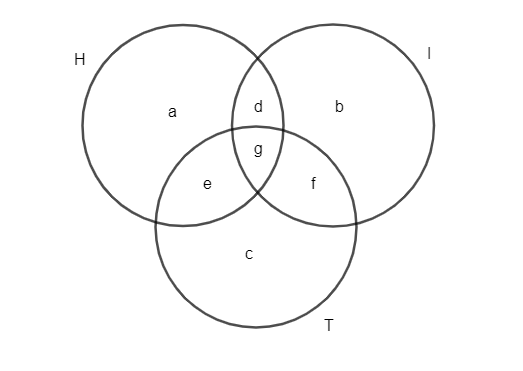Courses
Courses for Kids
Free study material
Free LIVE classes
MoreLIVE
Join Vedantu’s FREE Mastercalss

# A cement company earns a profit of Rs. 8 per bag of white cement sold and a loss of Rs. 5 per bag of grey cement sold. if the company sells 3000 bags of white cement and 5000 bags of grey cement in a month. What is its profit or loss?Verified
268.5k+ views
Hint: To solve the question, we have to calculate the total loss caused by selling grey cement and total profit earned by selling white cement by using the given information.

As per the given information, we have
The profit earned by a cement company by selling white cement = Rs.8 per bag.
The number of bags of white cement sold is equal to 3000 bags.
The total profit earned by selling the given number of bags$=3000\times 8=24,000$ rupees.
The loss by a cement company by selling grey cement = Rs.5 per bag.
The number of bags of white cement sold is equal to 5000 bags.
Therefore, the total loss by selling the given number of bags is,
$=5000\times 5=25,000$ rupees
Total loss is greater than total profit earned.25,000 > 24,000
Thus, loss occurs.
The total loss of the cement company = Total loss by selling the given number of bags - Total profit earned by selling the given number of bags.
Substituting the corresponding values, we get
Total loss = 25,000 – 24,000 = 1000
Thus, the total loss of the cement company is equal to Rs.1000.

Note: The possibility of mistake is to calculate loss without calculating the total cost using the number of bags of white and grey cement. The point to remember is loss is negative value and when total loss is greater than total profit then it is the case of loss for the cement company.
Last updated date: 27th Sep 2023
Total views: 268.5k
Views today: 4.68k